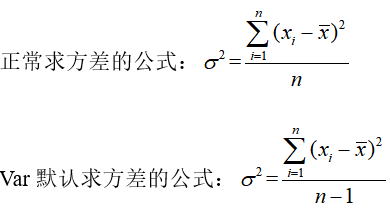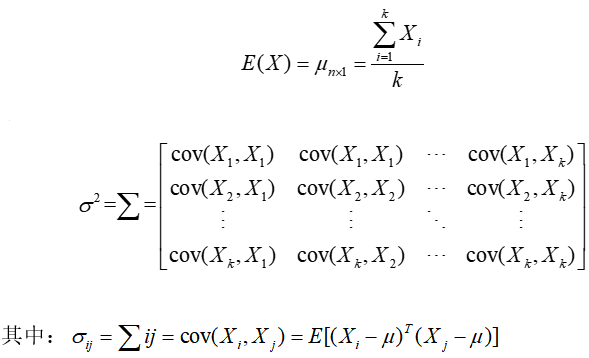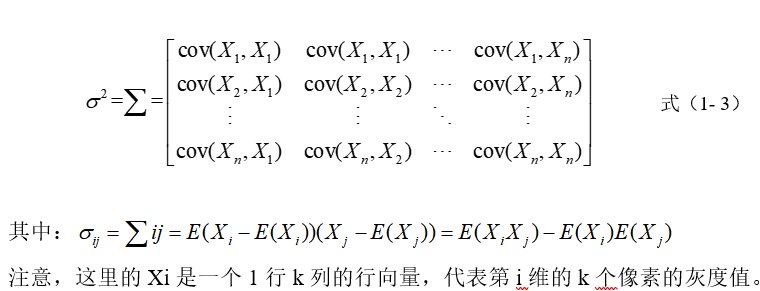• matlab求协方差矩阵>> A=[0,0,0;2,0,2;]A =0 0 02 0 2>> v = diag(cov(A))'v =2 0 2help里面的cov 函数，你自己看一下吧!MATLAB怎么计算协方差>> x=rand(1,5);>> y=2*rand(1,5);>> ...
matlab求协方差矩阵>> A=[0,0,0;2,0,2;]A =0 0 02 0 2>> v = diag(cov(A))'v =2 0 2help里面的cov 函数，你自己看一下吧!MATLAB 怎么计算协方差>> x=rand(1,5);>> y=2*rand(1,5);>> cov(x,y) %计算协方差ans =0.1079   -0.0225-0.0225    0.6148协方差分析是建立在方差分析和回归分析基础之上的一种统计分析方法。 方差分析是从质量因子的角度探讨因素不同水平对实验指标影响的差异。一般说来，质量因子是可以人为控制的。 回归分析是从数量因子的角度出发，通过建立回归方程来研究实验指标与一个(或几个)因子之间的数量关系。但大多数情况下，数量因子是不可以人为加以控制的。为什么考虑风险时，与市场组合的协方差更重要?组合的方差是有协方差矩阵的和决定的假如有N个资产构成一个组合那么协方差有N的平方减N个而方差只有N个所以前者重要这个告诉我们 股票的收益率看的是它对投资组合的风险贡献率 而不是自己的波动- -说得比较抽象Excel、Matlab的算出的协方差矩阵为什么不同大概是因为两种用的公式不一样。 因为你不可能根据样本得到协方差矩阵， 而只能根据样本做一个估计， 既然是估计那么当然有多种公式都是可行的， 而不能说哪一种就一定错【本文标题和网址】股票的协方差矩阵-matlab求协方差矩阵：http://www.jjta.cn/pzzx/160113.html声明本站分享的文章旨在促进信息交流，不以盈利为目的，本文观点与本站立场无关，不承担任何责任。如有伤害到您的利益，请联系站长删除！
展开全文• 介绍了Matlab 中如何使用 mean 、var 、std、cov 函数等
今天想用 Matlab 来算一个矩阵数据的均值和方差给我整迷了，好气！！所以做点笔记，以免下次在跳进坑里。

主博客：https://blog.csdn.net/Gou_Hailong/article/details/106092705

1.预备知识
关于算方差等，matlab提供了好几个函数：mean、var、std、cov，首先我们先看看这几个函数咋用的。
以下用到的矩阵都为：
X = [1 2 3; 3 3 6; 4 6 8; 4 7 7; 8 5 9]
=============================
X =

1     2     3
3     3     6
4     6     8
4     7     7
8     5     9

1.mean
/*这个函数还好理解，算平均值
如果参数是向量就直接是平均值
如果参数是矩阵，就会有俩种模式
1 返回一个行向量，计算各列的平均值（默认）
2 返回一个列向量，计算各行的平均值*/
>> mean(X(:,1))

ans =

4

>> mean(X(1,:))

ans =

2

>> mean(X)

ans =

4.0000    4.6000    6.6000

>> mean(X,2)

ans =

2.0000
4.0000
6.0000
6.0000
7.3333

如果一个矩阵中有nan的话，如何来求这个矩阵的均值呢？
AAt = reshape(AA, size(AA,1)*size(AA,2),1);
mean(AAt,'omitnan');

2.var
这个是用来算方差的，但是分母为（n-1），好像是为了搞成无偏估计，概率论的知识，忘了（😓）var(X,W,DIM)
/*这个函数求方差，公式参上，默认：var(X,0,1)
参数X为向量时：w=0:n-1  w=1:n
参数X为矩阵时：w=0:n-1  w=1:n
dim=1 返回一个行向量，计算各列的方差（默认）
dim=2 返回一个列向量，计算各行的方差
*/
>> var(X(1,:))

ans =

1

>> var(X(:,1))

ans =

6.5000

>> var(X,1,1)

ans =

5.2000    3.4400    4.2400

>> var(X,1,2)

ans =

0.6667
2.0000
2.6667
2.0000
2.8889

>> var(X,0,1)

ans =

6.5000    4.3000    5.3000

>> var(X,0,2)

ans =

1.0000
3.0000
4.0000
3.0000
4.3333

3.std
这个函数用来求标准差，就是var开方，调用形式和var类似都是var(X,W,DIM) 这里就不在赘述。
4.cov
我们先来回顾一下协方差矩阵是咋算的：
设X为一个向量，其均值表示为E(X)，那么它的方差为：D(X)=E((E(X)-Xi)^2)
这时，又有另一个向量Y(与X长度相同) ,其均值表示为E(Y)
那么它俩的协方差为：cov(X,Y)=E((E(X)-Xi)*(E(Y)-Yi)) = E(XY)－E(X)*E(Y)
协方差与方差有如下关系：
D(X+Y)=D(X)+D(Y)+2Cov(X，Y)
D(X-Y)=D(X)+D(Y)-2Cov(X，Y)
ok，接下来我们一起用matlab 来算算。
cov(X,Y,w)
/*用来计算向量X和Y 的协方差矩阵
没有Y时，用来算X的方差。
w=0:n-1  w=1:n  0 默认
当X是矩阵时：
算的是将每一列当做一个X(或Y),
X若为mxn 的矩阵，得到的是nxn的协方差矩阵*/
>> cov(X,1)

ans =

5.2000    2.2000    4.2000
2.2000    3.4400    2.8400
4.2000    2.8400    4.2400

>> cov(X,0)

ans =

6.5000    2.7500    5.2500
2.7500    4.3000    3.5500
5.2500    3.5500    5.3000

>> cov(X(:,1),X(:,2))

ans =

6.5000    2.7500
2.7500    4.3000

ok，至此已经介绍完了，下面的内容是我自己的思想斗争，大家可以忽略，忽略…
5.一个奇怪的东西
我现在突然意识到是我的指导思想错了，我写这篇博客的目的是为了得到一组遥感影像数据（某一类）的协方差矩阵，可现在发现一个cov 完全可以解决，之前想的是：其中n是n个波段，k是k个像素点，也就是说，每个像素点有n个灰度；也即有一组数据，有n个维度，这组数据共有k个。之后想求其协方差矩阵。
均值肯定是这k个样本点的均值，为一个n维的向量
从一维向量（行向量1xk）求方差 合理外延就会想到上述公式，但是这个并不是我所需要的，这个是一个kxk的矩阵，我想要的是一个nxn的矩阵，这两个东西到底是何含义，想到爆也想不出来（看来需要时间的积淀了！）
还是看个实例比较容易理解一点。
下面的实例是用来解决上面那个公式引申出来的。
2.实例
1.我们先建个矩阵
X = [1 2 3; 3 3 6; 4 6 8; 4 7 7; 8 5 9]
=============================
X =

1     2     3
3     3     6
4     6     8
4     7     7
8     5     9

如上，这是一个5行乘3列的矩阵，我们假设这个矩阵每一列代表遥感影像一个位置处的5个波段的灰度值，也就是说，有个遥感影像，他有三个点，5波段。我们想算他的均值和协方差矩阵，期望得到的均值是一个5行1列的列向量，希望得到的协方差矩阵是一个3行3列 （实际上是5x5）的矩阵。
我们还想得到一种协方差矩阵，它是5行5列的矩阵。2.为了验证，matlab 计算的正确性，我们先手动算一遍：
均值向量为：
mean=
2.0000
4.0000
6.0000
6.0000
7.3333

协方差矩阵1为：
cov=
10.4444     -2.5556     -7.8889
-2.5556      7.4444     -4.8889
-7.8889     -4.8889     12.7778

协方差矩阵2为：
cov=
10.4444     -2.5556     -7.8889
-2.5556      7.4444     -4.8889
-7.8889     -4.8889     12.7778

3.我们来看看用 matlab 自带的函数mean、cov算出来的都是啥：
>> mean(X,1)

ans =

4.0000    4.6000    6.6000

>> mean(X,2)%很遗憾，只有这一个达到了我们的期望

ans =

2.0000
4.0000
6.0000
6.0000
7.3333

>> cov(X)

ans =

6.5000    2.7500    5.2500
2.7500    4.3000    3.5500
5.2500    3.5500    5.3000

那我们将X转置一下在计算会不会好点呢？
>> X=X'

X =

1     3     4     4     8
2     3     6     7     5
3     6     8     7     9

>> mean(X,1)

ans =

2.0000    4.0000    6.0000    6.0000    7.3333

>> mean(X,2)

ans =

4.0000
4.6000
6.6000

>> cov(X)

ans =

1.0000    1.5000    2.0000    1.5000    0.5000
1.5000    3.0000    3.0000    1.5000    2.5000
2.0000    3.0000    4.0000    3.0000    1.0000
1.5000    1.5000    3.0000    3.0000   -1.0000
0.5000    2.5000    1.0000   -1.0000    4.3333

结果还是不符合我们的期望。这是为什么呢？原来这个Matlab计算协方差矩阵的时候，是用每一列作为一个元素（这与我们的期望相符）对角线的方差是计算这一列中的方差（就是其他的元素不考虑，算出这一列中的几个数的均值，之后在算这几个数的方差），非对角线咋算呢？非对角线列是相应列的协方差。
实在想不清楚，可以看看这篇文章：

https://blog.csdn.net/clam_clam/article/details/7335588

3.解决方案
OK，到目前为止，我们已经知道指望不上Matlab 自带的函数了（均值可以求，方差不行），那么我们就自食其力，自己写了个函数，用来计算上面想得到的方差。
%mycov.m  by@GHL
%此函数用来计算方差，
%m是一个n行，k列的矩阵
%每一个元素是m中的列向量，有n维
%m中共有k个元素
%最后得到的协方差矩阵是一个k行k列的矩阵
function sigma = mycov(m)%计算
X=mean(m,2);%均值
n=size(m,1);%n维
k=size(m,2);%k个元素
sigma=zeros(k);
for i = 1:k
for j = 1:k
sigma(i,j)=(m(:,i)-X)'*(m(:,j)-X);
end
end
return;
end

验证：
>> X=X'

X =

1     2     3
3     3     6
4     6     8
4     7     7
8     5     9

>> mycov(X)

ans =

10.4444   -2.5556   -7.8889
-2.5556    7.4444   -4.8889
-7.8889   -4.8889   12.7778

参考/引用 文章
 clam_clam-CSDN博主：https://blog.csdn.net/clam_clam/article/details/7335588


2020/6/16
【小感慨】
这么一个小玩意花了我一下午来捋清，好气！！差点爆粗口，真是用matlab 的基础还很不好，不管怎么说，这次终于搞清楚了（只差哪两个协方差矩阵没搞明白），希望下次不会在迷了😞😞😞


展开全文• X = [12.5 586;  24 754;  15.3 850;  18 667;  31.2 750];%X是初始矩阵 CovX=cov(X); CorrX=corr(X);
X = [12.5 586;
24 754;
15.3 850;
18 667;
31.2 750];%X是初始矩阵
CovX=cov(X);
CorrX=corr(X);

展开全文• ## matlab协方差矩阵

千次阅读 2018-05-29 15:56:28
参考自文章：http://blog.163.com/yuyang_tech/blog/static/216050083201382610424490/cov(X,Y) 求矩阵X与Y的协方差矩阵。若X大小为M*N，Y为K*P，则X，Y的大小必须满足M*N=K*P，即X，Y的元素个数相同。此时，cov(X,...
参考自文章：http://blog.163.com/yuyang_tech/blog/static/216050083201382610424490/cov(X,Y) 求矩阵X与Y的协方差矩阵。若X大小为M*N，Y为K*P，则X，Y的大小必须满足M*N=K*P，即X，Y的元素个数相同。此时，cov(X,Y) 等于cov([X(:) Y(:)])和cov(X(:),Y(:))，即计算两个向量的协方差矩阵，得到的结果为2*2矩阵。[S(X(:))	C(X(:),Y(:)); C(Y(:),X(:)) 	S(Y(:));]记录一下matlab中协方差矩阵的算法实际计算方法，一定要注意信号被降维了
展开全文• 本博文源于matlab基础，主要对协方差矩阵如何生成进行讲解。该函数使用randn cov使用 randn(m,n) % 生成一个m*n标准正态分布 矩阵 cov(m)% 出m的协方差矩阵 例子：出5*6阶服从正态分布随机数的协方差矩阵 >&...数学建模
• Covariance 翻译为协方差，因此，MATLAB里面的函数cov也就是求协方差了。至于MATLAB语言里面的协方差函数cov的语法是什么样的以及怎么用的，我们稍后再说，这里首先介绍下协方差相关的基础知识点。 本文内容参考自...
• 共回答了23个问题采纳率：95.7%Matlab函数：mean>>X=[1,2,3]>>mean(X)=2如果X是一个矩阵,则其均值是一个向量组.mean(X,1)为列向量的均值,mean(X,2)为行向量的均值.>>X=[1 2 34 5 6]>>mean(X...
• MATLAB中jacobian是用来计算Jacobi矩阵的函数 syms r l f x=r*cos(l)*cos(f); y=r*cos(l)*sin(f); z=r*sin(l); J=jacobian([x;y;z],[r l f]) 结果： J = [ cos(l)*cos(f), -r*sin(l)*cos(f), -r*cos(l)*sin(f...
• 求协方差矩阵matlab代码实现： % 计算矩阵协方差矩阵 % 加载数据 dataSet = [-1,1,0;-4,3,0;1,0,2]; %% 方法一：直接调用 dataCov = cov(dataSet); %% 方法二：了解原理，一步步计算 [rows, cols] = ...
• Matlab中cov函数详细解读 １、向量的方差与协方差矩阵 cov(x) 求向量x的方差。 cov(x)为一个数值，数值大小计算公式为S(x)。 ...２、矩阵协方差矩阵 cov(X) 求矩阵X的协方差矩阵。...
• 、 设X是n*p的data矩阵，n代表样本数，p...求协方差矩阵具体步骤： 先对每一列进行均值化操作，然后对均值化后的X, X'X即是协方差矩阵 如果是MAtlab的话， 直接调用cov(X)即可得到p*p的协方差矩阵
• 1、 均值数学定义：Matlab函数：mean>>X=[1,2,3]>>mean(X)=2如果X是一个矩阵，则其均值是一个向量组。mean(X,1)为列向量的均值，mean(X,2)为行向量的均值。>>X=[1 2 34 5 6]>>mean(X,1)=...
• 出图像矩阵所有数值的均值。 注意点： ①图像矩阵的每个值都是uint8类型的，uint8的范围是0-255，在进行求和之前需要把图像矩阵转换成double类型，如果不转换，继续对uint8类型进行运算会产生溢出； ②将矩阵变为...
• 1、均值数学定义：Matlab函数：mean>>X=[1,2,3]>>mean(X)=2如果X是一个矩阵，则其均值是一个向量组。mean(X,1)为列向量的均值，mean(X,2)为行向量的均值。>>X=[1 2 34 5 6]>>mean(X,1)=[2.5......

# matlab求矩阵协方差matlab 订阅